## List of Deep Learning Layers

To learn how to create networks from layers for different tasks, see the following examples.

Create deep learning networks for image classification or regression.
Create deep learning networks for sequence and time series data.
Create deep learning network for audio data.Speech Command Recognition Using Deep Learning
Create deep learning network for text data.

### Deep Learning Layers

Use the following functions to create different layer types. Alternatively, use the Deep Network Designer app to create networks interactively.

To learn how to define your own custom layers, see Define Custom Deep Learning Layers.

#### Input Layers

LayerDescription`imageInputLayer`

An image input layer inputs 2-D images to a network and applies data normalization.`image3dInputLayer`

A 3-D image input layer inputs 3-D images or volumes to a network and applies data normalization.`sequenceInputLayer`

A sequence input layer inputs sequence data to a network.`featureInputLayer`

A feature input layer inputs feature data to a network and applies data normalization. Use this layer when you have a data set of numeric scalars representing features (data without spatial or time dimensions).`roiInputLayer` (Computer Vision Toolbox)

An ROI input layer inputs images to a Fast R-CNN object detection network.

#### Convolution and Fully Connected Layers

LayerDescription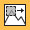`convolution2dLayer`

A 2-D convolutional layer applies sliding convolutional filters to the input.`convolution3dLayer`

A 3-D convolutional layer applies sliding cuboidal convolution filters to three-dimensional input.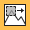`groupedConvolution2dLayer`

A 2-D grouped convolutional layer separates the input channels into groups and applies sliding convolutional filters. Use grouped convolutional layers for channel-wise separable (also known as depth-wise separable) convolution.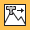`transposedConv2dLayer`

A transposed 2-D convolution layer upsamples feature maps.`transposedConv3dLayer`

A transposed 3-D convolution layer upsamples three-dimensional feature maps.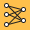`fullyConnectedLayer`

A fully connected layer multiplies the input by a weight matrix and then adds a bias vector.

#### Sequence Layers

LayerDescription`sequenceInputLayer`

A sequence input layer inputs sequence data to a network.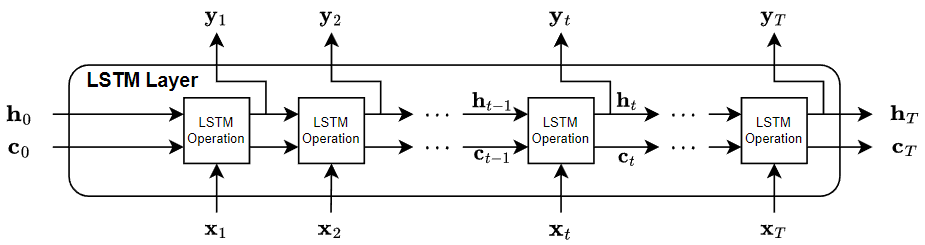`lstmLayer`

An LSTM layer learns long-term dependencies between time steps in time series and sequence data.`bilstmLayer`

A bidirectional LSTM (BiLSTM) layer learns bidirectional long-term dependencies between time steps of time series or sequence data. These dependencies can be useful when you want the network to learn from the complete time series at each time step.`gruLayer`

A GRU layer learns dependencies between time steps in time series and sequence data.`sequenceFoldingLayer`

A sequence folding layer converts a batch of image sequences to a batch of images. Use a sequence folding layer to perform convolution operations on time steps of image sequences independently.`sequenceUnfoldingLayer`

A sequence unfolding layer restores the sequence structure of the input data after sequence folding.`flattenLayer`

A flatten layer collapses the spatial dimensions of the input into the channel dimension.`wordEmbeddingLayer` (Text Analytics Toolbox)

A word embedding layer maps word indices to vectors.

#### Activation Layers

LayerDescription`reluLayer`

A ReLU layer performs a threshold operation to each element of the input, where any value less than zero is set to zero.`leakyReluLayer`

A leaky ReLU layer performs a threshold operation, where any input value less than zero is multiplied by a fixed scalar.`clippedReluLayer`

A clipped ReLU layer performs a threshold operation, where any input value less than zero is set to zero and any value above the clipping ceiling is set to that clipping ceiling.`eluLayer`

An ELU activation layer performs the identity operation on positive inputs and an exponential nonlinearity on negative inputs.`tanhLayer`

A hyperbolic tangent (tanh) activation layer applies the tanh function on the layer inputs.`swishLayer`

A swish activation layer applies the swish function on the layer inputs.`preluLayer` (Custom layer example)

A PReLU layer performs a threshold operation, where for each channel, any input value less than zero is multiplied by a scalar learned at training time.

#### Normalization, Dropout, and Cropping Layers

LayerDescription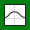`batchNormalizationLayer`

A batch normalization layer normalizes a mini-batch of data across all observations for each channel independently. To speed up training of the convolutional neural network and reduce the sensitivity to network initialization, use batch normalization layers between convolutional layers and nonlinearities, such as ReLU layers.`groupNormalizationLayer`

A group normalization layer normalizes a mini-batch of data across grouped subsets of channels for each observation independently. To speed up training of the convolutional neural network and reduce the sensitivity to network initialization, use group normalization layers between convolutional layers and nonlinearities, such as ReLU layers.`instanceNormalizationLayer`

An instance normalization layer normalizes a mini-batch of data across each channel for each observation independently. To improve the convergence of training the convolutional neural network and reduce the sensitivity to network hyperparameters, use instance normalization layers between convolutional layers and nonlinearities, such as ReLU layers.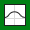`layerNormalizationLayer`

A layer normalization layer normalizes a mini-batch of data across all channels for each observation independently. To speed up training of recurrent and multi-layer perceptron neural networks and reduce the sensitivity to network initialization, use layer normalization layers after the learnable layers, such as LSTM and fully connected layers.`crossChannelNormalizationLayer`

A channel-wise local response (cross-channel) normalization layer carries out channel-wise normalization.`dropoutLayer`

A dropout layer randomly sets input elements to zero with a given probability.`crop2dLayer`

A 2-D crop layer applies 2-D cropping to the input.`crop3dLayer`

A 3-D crop layer crops a 3-D volume to the size of the input feature map.`resize2dLayer` (Image Processing Toolbox)

A 2-D resize layer resizes 2-D input by a scale factor, to a specified height and width, or to the size of a reference input feature map.`resize3dLayer` (Image Processing Toolbox)

A 3-D resize layer resizes 3-D input by a scale factor, to a specified height, width, and depth, or to the size of a reference input feature map.

#### Pooling and Unpooling Layers

LayerDescription`averagePooling2dLayer`

An average pooling layer performs downsampling by dividing the input into rectangular pooling regions and computing the average values of each region.`averagePooling3dLayer`

A 3-D average pooling layer performs downsampling by dividing three-dimensional input into cuboidal pooling regions and computing the average values of each region.`globalAveragePooling2dLayer`

A global average pooling layer performs downsampling by computing the mean of the height and width dimensions of the input.`globalAveragePooling3dLayer`

A 3-D global average pooling layer performs downsampling by computing the mean of the height, width, and depth dimensions of the input.`maxPooling2dLayer`

A max pooling layer performs downsampling by dividing the input into rectangular pooling regions, and computing the maximum of each region.`maxPooling3dLayer`

A 3-D max pooling layer performs downsampling by dividing three-dimensional input into cuboidal pooling regions, and computing the maximum of each region.`globalMaxPooling2dLayer`

A global max pooling layer performs downsampling by computing the maximum of the height and width dimensions of the input.`globalMaxPooling3dLayer`

A 3-D global max pooling layer performs downsampling by computing the maximum of the height, width, and depth dimensions of the input.`maxUnpooling2dLayer`

A max unpooling layer unpools the output of a max pooling layer.

#### Combination Layers

LayerDescription`additionLayer``multiplicationLayer`

A multiplication layer multiplies inputs from multiple neural network layers element-wise.`depthConcatenationLayer`

A depth concatenation layer takes inputs that have the same height and width and concatenates them along the third dimension (the channel dimension).`concatenationLayer`

A concatenation layer takes inputs and concatenates them along a specified dimension. The inputs must have the same size in all dimensions except the concatenation dimension.`weightedAdditionLayer` (Custom layer example)

A weighted addition layer scales and adds inputs from multiple neural network layers element-wise.

#### Object Detection Layers

LayerDescription`roiInputLayer` (Computer Vision Toolbox)

An ROI input layer inputs images to a Fast R-CNN object detection network.`roiMaxPooling2dLayer` (Computer Vision Toolbox)

An ROI max pooling layer outputs fixed size feature maps for every rectangular ROI within the input feature map. Use this layer to create a Fast or Faster R-CNN object detection network.`roiAlignLayer` (Computer Vision Toolbox)

An ROI align layer outputs fixed size feature maps for every rectangular ROI within an input feature map. Use this layer to create a Mask-RCNN network.`anchorBoxLayer` (Computer Vision Toolbox)

An anchor box layer stores anchor boxes for a feature map used in object detection networks.`regionProposalLayer` (Computer Vision Toolbox)

A region proposal layer outputs bounding boxes around potential objects in an image as part of the region proposal network (RPN) within Faster R-CNN.`ssdMergeLayer` (Computer Vision Toolbox)

An SSD merge layer merges the outputs of feature maps for subsequent regression and classification loss computation.`spaceToDepthLayer` (Image Processing Toolbox)

A space to depth layer permutes the spatial blocks of the input into the depth dimension. Use this layer when you need to combine feature maps of different size without discarding any feature data.`depthToSpace2dLayer` (Image Processing Toolbox)

A 2-D depth to space layer permutes data from the depth dimension into blocks of 2-D spatial data.`rpnSoftmaxLayer` (Computer Vision Toolbox)

A region proposal network (RPN) softmax layer applies a softmax activation function to the input. Use this layer to create a Faster R-CNN object detection network.`focalLossLayer` (Computer Vision Toolbox)

A focal loss layer predicts object classes using focal loss.`rpnClassificationLayer` (Computer Vision Toolbox)

A region proposal network (RPN) classification layer classifies image regions as either object or background by using a cross entropy loss function. Use this layer to create a Faster R-CNN object detection network.`rcnnBoxRegressionLayer` (Computer Vision Toolbox)

A box regression layer refines bounding box locations by using a smooth L1 loss function. Use this layer to create a Fast or Faster R-CNN object detection network.

LayerDescription`projectAndReshapeLayer` (Custom layer example)

A project and reshape layer takes as input 1-by-1-by-`numLatentInputs` arrays and converts them to images of the specified size. Use project and reshape layers to reshape the noise input to GANs.`embedAndReshapeLayer` (Custom layer example)

An embed and reshape layer takes as input numeric indices of categorical elements and converts them to images of the specified size. Use embed and reshape layers to input categorical data into conditional GANs.

#### Output Layers

LayerDescription`softmaxLayer`

A softmax layer applies a softmax function to the input.`sigmoidLayer`

A sigmoid layer applies a sigmoid function to the input such that the output is bounded in the interval (0,1).`classificationLayer`

A classification layer computes the cross-entropy loss for classification and weighted classification tasks with mutually exclusive classes.`regressionLayer`

A regression layer computes the half-mean-squared-error loss for regression tasks.`pixelClassificationLayer` (Computer Vision Toolbox)

A pixel classification layer provides a categorical label for each image pixel or voxel.`dicePixelClassificationLayer` (Computer Vision Toolbox)

A Dice pixel classification layer provides a categorical label for each image pixel or voxel using generalized Dice loss.`focalLossLayer` (Computer Vision Toolbox)

A focal loss layer predicts object classes using focal loss.`rpnSoftmaxLayer` (Computer Vision Toolbox)

A region proposal network (RPN) softmax layer applies a softmax activation function to the input. Use this layer to create a Faster R-CNN object detection network.`rpnClassificationLayer` (Computer Vision Toolbox)

A region proposal network (RPN) classification layer classifies image regions as either object or background by using a cross entropy loss function. Use this layer to create a Faster R-CNN object detection network.`rcnnBoxRegressionLayer` (Computer Vision Toolbox)

A box regression layer refines bounding box locations by using a smooth L1 loss function. Use this layer to create a Fast or Faster R-CNN object detection network.`tverskyPixelClassificationLayer` (Custom layer example)

A Tversky pixel classification layer provides a categorical label for each image pixel or voxel using Tversky loss.`sseClassificationLayer` (Custom layer example)

A classification SSE layer computes the sum of squares error loss for classification problems.`maeRegressionLayer` (Custom layer example)

A regression MAE layer computes the mean absolute error loss for regression problems.